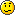Page 1 of 1

### Calculating light Speed

Posted: Thu Jan 10, 2013 2:14 pm
I am calculating the light speed through different densities of jello using a laser. I found out much information about Snell's Law and the Index of Refraction. I know that I will need to measure the angle of refraction of the beam once it enters the jello in comparison to the normal. I'm sure there is a way I can enter that measurement into the formula in the place of θ1, but I wasn't sure what I would replace the other variables with or what additional measurements I need to take to figure out how fast the laser is going through the different densities of jello. My final measurements should be the speed of light through the different densities of jello, but how do I get light speed out of these two formulas?Snell's Law: n1sinθ1= n2sinθ2 (the 1 and 2 after n and θ are subscripts)
n1= the index of refraction of the meium in which the incident ray travels
θ1= the angle
Index of Refraction: n=c/v
n= the index of refraction
v= the velocity of light in the medium
c= the velocity of light in a vacuum

### Re: Calculating light Speed

Posted: Sun Jan 13, 2013 12:01 pm
Hello,

I am assuming that you are following the experiment given here
http://www.sciencebuddies.org/science-fair-projects/project_ideas/Phys_p009.shtml#procedure

If you are and you have the correct measurements for the angles then this really boils down to a simple problem of substituting in the correct values of the different variables into Snell's Law.

What you have to do is to recognize that the laser is passing through two mediums i.e. air and jello (we will ignore the plastic container unless you made the jello thick enough to stand on its own in which case you don't need the container).

Now Snell's Law really boils down to the algebra because we just need to find one variable i.e. the speed of light in jello.

You did correctly state Snell's Law and the form you will need it in is
Velocity in jello = [sin(refracted angle in jello)] /[sin(incident angle in air)]* (velocity in air)

This comes from doing a little bit of derivation from the original Snell's Law and recognizing that the refractive index is the ratio of the velocity of light in the medium to the velocity of light in vacuum (which is a universal constant). I encourage you to do that bit of math and find out for yourself.

Now your measurements will give you the refracted and the incident angles and you can look up the velocity of light in air at different temperatures online or in a book. With that information you should have no trouble repeating this experiment multiple times with jello's of different density and finding the relationship between density of the medium and the speed of light in that medium.

Also, by your post I get the feeling that you are a little confused about the incident and the refracted angles so let me clear that for you if it isn't clear. The incident angle is the angle the laser makes with the normal to the interface in the air while the refracted angle is the angle the laser makes with the same normal as before in the jello.

Hope this helps and let me know if you have any more questions.